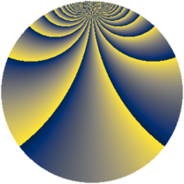# Properties

 Label 729.2.iLevel $729$ Weight $2$ Character orbit 729.i Rep. character $\chi_{729}(10,\cdot)$ Character field $\Q(\zeta_{81})$ Dimension $1404$ Newform subspaces $1$ Sturm bound $162$ Trace bound $0$

# Related objects

## Defining parameters

 Level: $$N$$ $$=$$ $$729 = 3^{6}$$ Weight: $$k$$ $$=$$ $$2$$ Character orbit: $$[\chi]$$ $$=$$ 729.i (of order $$81$$ and degree $$54$$) Character conductor: $$\operatorname{cond}(\chi)$$ $$=$$ $$243$$ Character field: $$\Q(\zeta_{81})$$ Newform subspaces: $$1$$ Sturm bound: $$162$$ Trace bound: $$0$$

## Dimensions

The following table gives the dimensions of various subspaces of $$M_{2}(729, [\chi])$$.

Total New Old
Modular forms 4536 1512 3024
Cusp forms 4212 1404 2808
Eisenstein series 324 108 216

## Trace form

 $$1404 q + 54 q^{2} - 54 q^{4} + 54 q^{5} - 54 q^{7} + 54 q^{8} + O(q^{10})$$ $$1404 q + 54 q^{2} - 54 q^{4} + 54 q^{5} - 54 q^{7} + 54 q^{8} - 54 q^{10} + 54 q^{11} - 54 q^{13} + 54 q^{14} - 54 q^{16} + 54 q^{17} - 54 q^{19} + 54 q^{20} - 54 q^{22} + 54 q^{23} - 54 q^{25} + 54 q^{26} - 54 q^{28} + 54 q^{29} - 54 q^{31} + 54 q^{32} - 54 q^{34} + 54 q^{35} - 54 q^{37} + 54 q^{38} - 54 q^{40} + 54 q^{41} - 54 q^{43} + 54 q^{44} - 54 q^{46} + 54 q^{47} - 54 q^{49} + 54 q^{50} - 54 q^{52} + 54 q^{53} - 54 q^{55} + 54 q^{56} - 54 q^{58} + 54 q^{59} - 54 q^{61} + 54 q^{62} - 54 q^{64} - 54 q^{67} - 135 q^{68} - 54 q^{70} - 54 q^{71} - 54 q^{73} - 162 q^{74} - 54 q^{76} - 162 q^{77} - 54 q^{79} - 351 q^{80} - 27 q^{82} - 54 q^{83} - 54 q^{85} - 162 q^{86} - 54 q^{88} - 81 q^{89} - 54 q^{91} - 270 q^{92} - 54 q^{94} - 54 q^{95} - 54 q^{97} - 54 q^{98} + O(q^{100})$$

## Decomposition of $$S_{2}^{\mathrm{new}}(729, [\chi])$$ into newform subspaces

Label Dim $A$ Field CM Traces $q$-expansion
$a_{2}$ $a_{3}$ $a_{5}$ $a_{7}$
729.2.i.a $1404$ $5.821$ None $$54$$ $$0$$ $$54$$ $$-54$$

## Decomposition of $$S_{2}^{\mathrm{old}}(729, [\chi])$$ into lower level spaces

$$S_{2}^{\mathrm{old}}(729, [\chi]) \cong$$ $$S_{2}^{\mathrm{new}}(243, [\chi])$$$$^{\oplus 2}$$